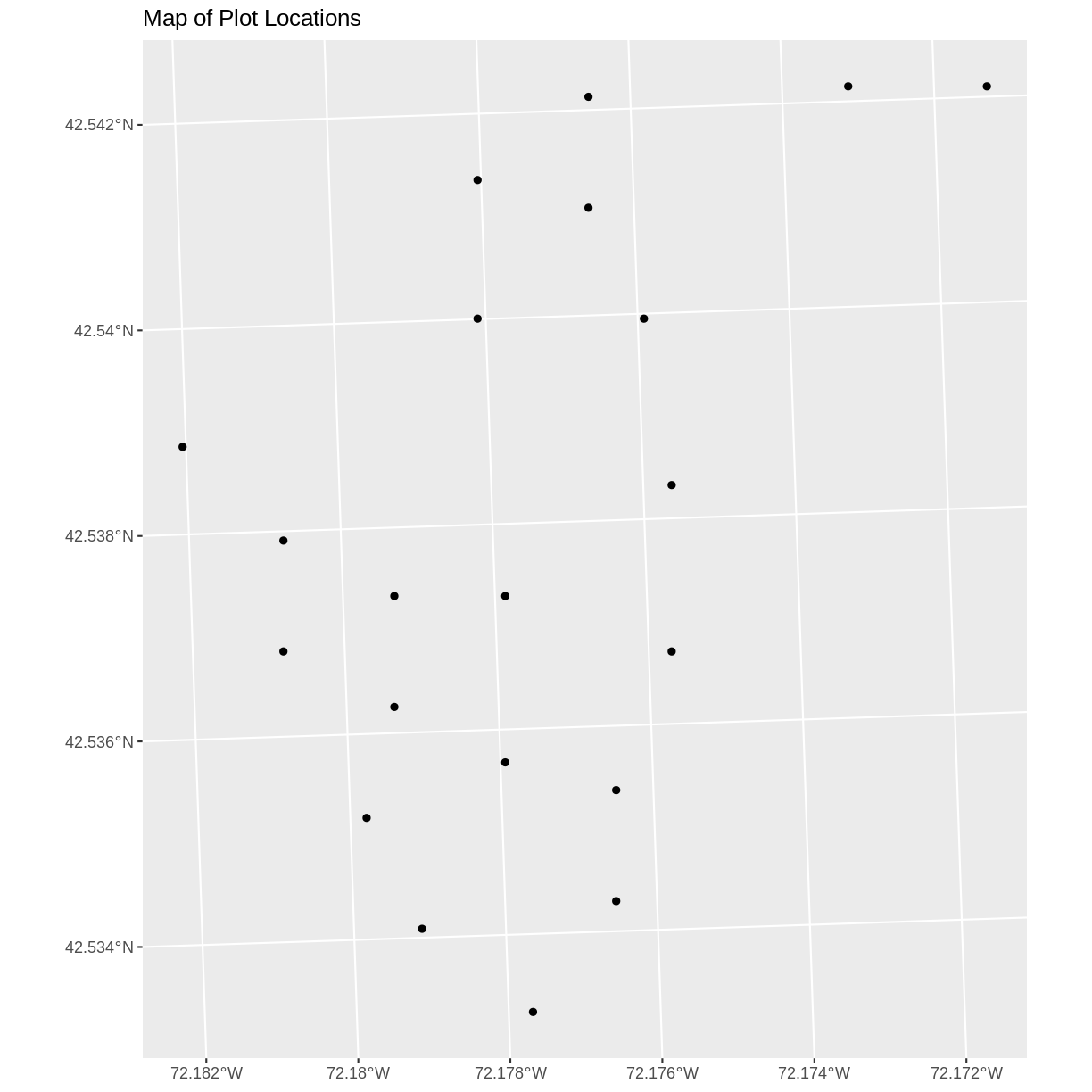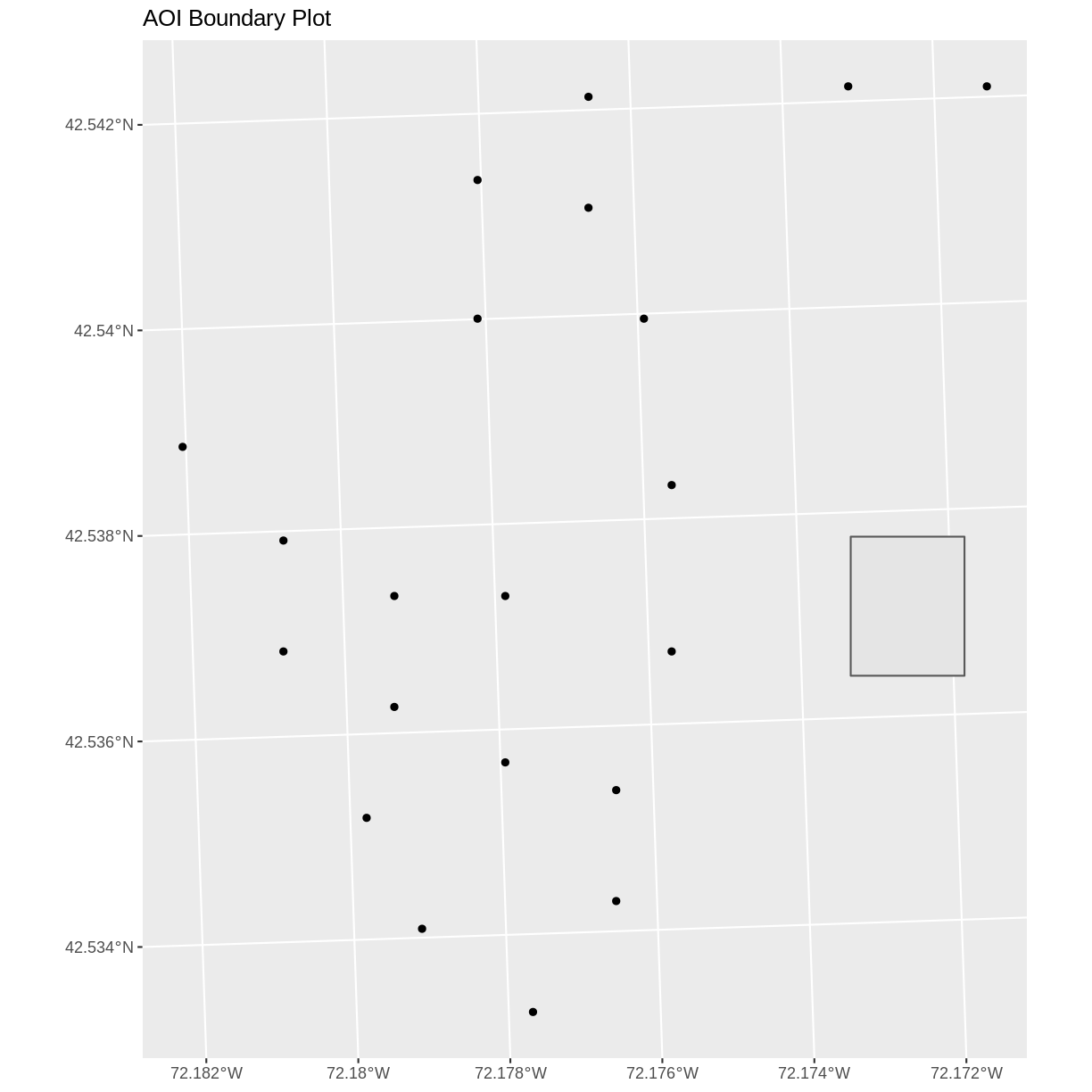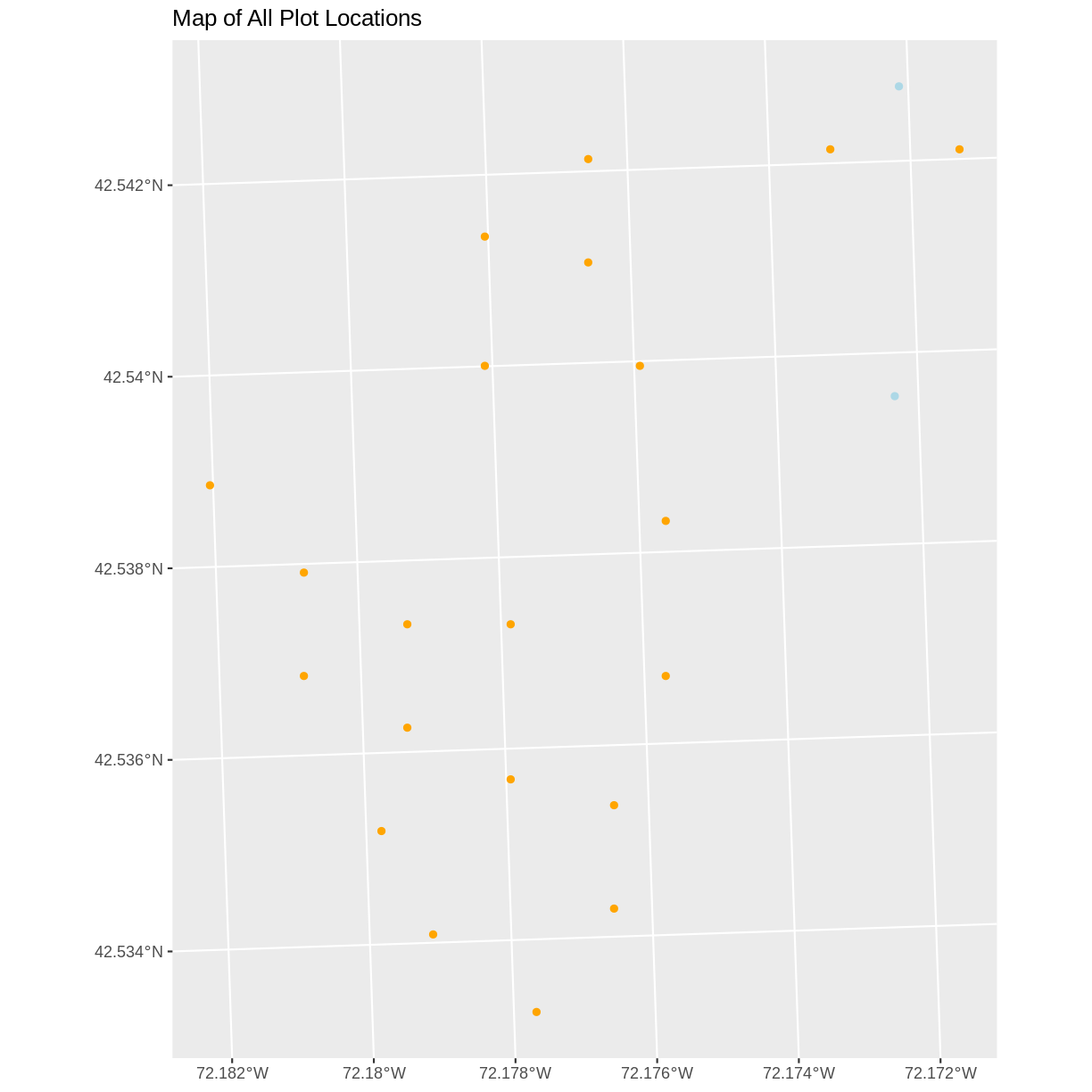This lesson is being piloted (Beta version)

# Convert from .csv to a Shapefile in R

## Overview

Teaching: 40 min
Exercises: 20 min
Questions
• How can I import CSV files as shapefiles in R?

Objectives
• Import .csv files containing x,y coordinate locations into R as a data frame.

• Convert a data frame to a spatial object.

• Export a spatial object to a text file.

## Things You’ll Need To Complete This Episode

See the lesson homepage for detailed information about the software, data, and other prerequisites you will need to work through the examples in this episode.

This episode will introduce how to import spatial points stored in `.csv` (Comma Separated Value) format into R as an `sf` spatial object.

We will continue to work with specific packages for working with spatial data. Let’s load all of the packages we have been working with in this lesson.

``````#library(rgdal)
library(ggplot2)
library(dplyr)
library(sf)
``````

We will also continue to work with the HARV vector data. You may have these layers already loaded into your enviroment, but if not, load them now.

``````# Learners will have this data loaded from earlier episodes
aoi_boundary_HARV <- st_read("data/vector/HarClip_UTMZ18.shp")
lines_HARV <- st_read("data/vector/HARV_roads.shp")
point_HARV <- st_read("data/vector/HARVtower_UTM18N.shp")
``````

## Spatial Data in Text Format

Spatial data are sometimes stored in a text file format (`.txt` or `.csv`). The data we downloaded includes a text file called `HARV_PlotLocations.csv`. This file contains `x, y` (point) locations for study plot where NEON collects data on vegetation and other ecological metrics.

While it is very common to store vector data as an Esri shapefile format and most programs can create and read that format, you may encounter data stored in a text file. Text files can be opened in any program and can be converted to any type of vector file format, so it is often useful for long-term storate and sharing with others who may not be familiar with the `shapefile` format.

We would like to:

• Import the `.csv` file and convert to a spatial object.
• Create a map of the plot locations.
• Export the data in a `shapefile` format to share with our colleagues. This shapefile can be imported into any GIS software.

If the text file has an associated `x` and `y` location column, then we can convert it into an `sf` spatial object. The `sf` object allows us to store both the `x,y` values that represent the coordinate location of each point and the associated attribute data - or columns describing each feature in the spatial object.

## Import .csv

To begin let’s import the `HARV_PlotLoations.csv` file with the locations at the NEON Harvard Forest Field Site. Once we import the file, we can check to see if the file contains plot coordinate as `x, y` attributes.

``````plot_locations_HARV <- read.csv("data/HARV_PlotLocations.csv")

str(plot_locations_HARV)
``````
``````'data.frame':	21 obs. of  16 variables:
\$ easting   : num  731405 731934 731754 731724 732125 ...
\$ northing  : num  4713456 4713415 4713115 4713595 4713846 ...
\$ geodeticDa: chr  "WGS84" "WGS84" "WGS84" "WGS84" ...
\$ utmZone   : chr  "18N" "18N" "18N" "18N" ...
\$ plotID    : chr  "HARV_015" "HARV_033" "HARV_034" "HARV_035" ...
\$ stateProvi: chr  "MA" "MA" "MA" "MA" ...
\$ county    : chr  "Worcester" "Worcester" "Worcester" "Worcester" ...
\$ domainName: chr  "Northeast" "Northeast" "Northeast" "Northeast" ...
\$ domainID  : chr  "D01" "D01" "D01" "D01" ...
\$ siteID    : chr  "HARV" "HARV" "HARV" "HARV" ...
\$ plotType  : chr  "distributed" "tower" "tower" "tower" ...
\$ subtype   : chr  "basePlot" "basePlot" "basePlot" "basePlot" ...
\$ plotSize  : int  1600 1600 1600 1600 1600 1600 1600 1600 1600 1600 ...
\$ elevation : num  332 342 348 334 353 ...
\$ soilTypeOr: chr  "Inceptisols" "Inceptisols" "Inceptisols" "Histosols" ...
\$ plotdim_m : int  40 40 40 40 40 40 40 40 40 40 ...
``````

We now have a data frame that contains 21 locations (rows) and 16 variables (attributes). Note that all of our character data was imported into R as factor (categorical) data. Next, let’s explore the dataframe to determine whether it contains columns with coordinate values. If we are lucky, our `.csv` will contain columns with the X and Y locations. We can look for attribute names such as:

• “X” and “Y” OR
• Latitude and Longitude OR
• easting and northing (UTM coordinates)

Let’s check out the column names of our dataframe.

``````names(plot_locations_HARV)
``````
``````  "easting"    "northing"   "geodeticDa" "utmZone"    "plotID"
 "stateProvi" "county"     "domainName" "domainID"   "siteID"
 "plotType"   "subtype"    "plotSize"   "elevation"  "soilTypeOr"
 "plotdim_m"
``````

## Identify X,Y Location Columns

Our column names include several fields that might contain spatial information. The `plot_locations_HARV\$easting` and `plot_locations_HARV\$northing` columns contain coordinate values. We can confirm this by looking at the first six rows of our data.

``````head(plot_locations_HARV\$easting)
``````
`````` 731405.3 731934.3 731754.3 731724.3 732125.3 731634.3
``````
``````head(plot_locations_HARV\$northing)
``````
`````` 4713456 4713415 4713115 4713595 4713846 4713295
``````

We have coordinate values in our data frame. In order to convert our data frame to an `sf` object, we also need to know the CRS associated with those coordinate values.

There are several ways to figure out the CRS of spatial data in text format.

1. We can check the file metadata in hopes that the CRS was recorded in the data.
2. We can explore the file itself to see if CRS information is embedded in the file header or somewhere in the data columns.

Following the `easting` and `northing` columns, there is a `geodeticDa` and a `utmZone` column. These appear to contain CRS information (`datum` and `projection`). Let’s view those next.

``````head(plot_locations_HARV\$geodeticDa)
``````
`````` "WGS84" "WGS84" "WGS84" "WGS84" "WGS84" "WGS84"
``````
``````head(plot_locations_HARV\$utmZone)
``````
`````` "18N" "18N" "18N" "18N" "18N" "18N"
``````

It is not typical to store CRS information in a column. But this particular file contains CRS information this way. The `geodeticDa` and `utmZone` columns contain the information that helps us determine the CRS:

• `geodeticDa`: WGS84 – this is geodetic datum WGS84
• `utmZone`: 18

In When Vector Data Don’t Line Up - Handling Spatial Projection & CRS in R we learned about the components of a `proj4` string. We need to know the datum, the projection, and in some cases a zone. For these data we know the datum is `WGS84`. While the projection is not specified as a column, we are given information about a UTM Zone. This means that our projection is `UTM`. Therefore, we have everything we need to assign a CRS to our data frame.

To create the `proj4` associated with UTM Zone 18 WGS84 we can look up the projection on the Spatial Reference website, which contains a list of CRS formats for each projection. From here, we can extract the proj4 string for UTM Zone 18N WGS84.

However, if we have other data in the UTM Zone 18N projection, it’s much easier to use the `st_crs()` function to extract the CRS in `proj4` format from that object and assign it to our new spatial object.

We’ve seen this CRS before with our Harvard Forest study site (`point_HARV`).

``````st_crs(point_HARV)
``````
``````Coordinate Reference System:
User input: 32618
wkt:
PROJCS["WGS_1984_UTM_Zone_18N",
GEOGCS["GCS_WGS_1984",
DATUM["WGS_1984",
SPHEROID["WGS_84",6378137,298.257223563]],
PRIMEM["Greenwich",0],
UNIT["Degree",0.017453292519943295],
AUTHORITY["EPSG","4326"]],
PROJECTION["Transverse_Mercator"],
PARAMETER["latitude_of_origin",0],
PARAMETER["central_meridian",-75],
PARAMETER["scale_factor",0.9996],
PARAMETER["false_easting",500000],
PARAMETER["false_northing",0],
UNIT["Meter",1],
AUTHORITY["EPSG","32618"]]
``````

The output above shows that the points shapefile is in UTM zone 18N. We can thus use the CRS from that spatial object to convert our non-spatial dataframe into an `sf` object.

Next, let’s create a `crs` object that we can use to define the CRS of our `sf` object when we create it.

``````utm18nCRS <- st_crs(point_HARV)
utm18nCRS
``````
``````Coordinate Reference System:
User input: 32618
wkt:
PROJCS["WGS_1984_UTM_Zone_18N",
GEOGCS["GCS_WGS_1984",
DATUM["WGS_1984",
SPHEROID["WGS_84",6378137,298.257223563]],
PRIMEM["Greenwich",0],
UNIT["Degree",0.017453292519943295],
AUTHORITY["EPSG","4326"]],
PROJECTION["Transverse_Mercator"],
PARAMETER["latitude_of_origin",0],
PARAMETER["central_meridian",-75],
PARAMETER["scale_factor",0.9996],
PARAMETER["false_easting",500000],
PARAMETER["false_northing",0],
UNIT["Meter",1],
AUTHORITY["EPSG","32618"]]
``````
``````class(utm18nCRS)
``````
`````` "crs"
``````

## .csv to sf object

Next, let’s convert our dataframe into an `sf` object. To do this, we need to specify:

1. The columns containing X (`easting`) and Y (`northing`) coordinate values
2. The CRS that the column coordinate represent (units are included in the CRS) - stored in our `utmCRS` object.

We will use the `st_as_sf()` function to perform the conversion. This function requires the layer to convert, the `X` and `Y` locations, and the projection information.

``````plot_locations_sp_HARV <- st_as_sf(plot_locations_HARV, coords = c("easting", "northing"), crs = utm18nCRS)
``````

We should double check the CRS to make sure it is correct.

``````st_crs(plot_locations_sp_HARV)
``````
``````Coordinate Reference System:
User input: 32618
wkt:
PROJCS["WGS_1984_UTM_Zone_18N",
GEOGCS["GCS_WGS_1984",
DATUM["WGS_1984",
SPHEROID["WGS_84",6378137,298.257223563]],
PRIMEM["Greenwich",0],
UNIT["Degree",0.017453292519943295],
AUTHORITY["EPSG","4326"]],
PROJECTION["Transverse_Mercator"],
PARAMETER["latitude_of_origin",0],
PARAMETER["central_meridian",-75],
PARAMETER["scale_factor",0.9996],
PARAMETER["false_easting",500000],
PARAMETER["false_northing",0],
UNIT["Meter",1],
AUTHORITY["EPSG","32618"]]
``````
``````class(plot_locations_sp_HARV)
``````
`````` "sf"         "data.frame"
``````

## Plot Spatial Object

We now have a spatial R object, we can plot our newly created spatial object.

``````ggplot() +
geom_sf(data = plot_locations_sp_HARV) +
ggtitle("Map of Plot Locations")
``````## Plot Extent

In Open and Plot Shapefiles in R we learned about spatial object extent. When we plot several spatial layers in R using `ggplot`, all of the layers of the plot are considered in setting the boundaries of the plot. To show this, let’s plot our `aoi_boundary_HARV` object with our vegetation plots.

``````ggplot() +
geom_sf(data = aoi_boundary_HARV) +
geom_sf(data = plot_locations_sp_HARV) +
ggtitle("AOI Boundary Plot")
``````When we plot the two layers together, `ggplot` sets the plot boundaries so that they are large enough to include all of the data included in all of the layers. That’s really handy!

## Export a Shapefile

We can write an R spatial object to a shapefile using the `st_write` function in `sf`. To do this we need the following arguments:

• the name of the spatial object (`plot_locations_sp_HARV`)
• the directory where we want to save our shapefile (to use `current = getwd()` or you can specify a different path)
• the name of the new shapefile (`PlotLocations_HARV`)
• the driver which specifies the file format (ESRI Shapefile)

We can now export the spatial object as a shapefile.

``````st_write(plot_locations_sp_HARV,
"data/PlotLocations_HARV.shp", driver = "ESRI Shapefile")
``````

## Challenge - Import & Plot Additional Points

We want to add two phenology plots to our existing map of vegetation plot locations.

Import the .csv: `HARV/HARV_2NewPhenPlots.csv` into R and do the following:

1. Find the X and Y coordinate locations. Which value is X and which value is Y?
2. These data were collected in a geographic coordinate system (WGS84). Convert the dataframe into an `sf` object.
3. Plot the new points with the plot location points from above. Be sure to add a legend. Use a different symbol for the 2 new points!

If you have extra time, feel free to add roads and other layers to your map!

## Answers

1) First we will read in the new csv file and look at the data structure.

``````newplot_locations_HARV <-
read.csv("data/HARV_2NewPhenPlots.csv")
str(newplot_locations_HARV)
``````
``````'data.frame':	2 obs. of  13 variables:
\$ decimalLat: num  42.5 42.5
\$ decimalLon: num  -72.2 -72.2
\$ country   : chr  "unitedStates" "unitedStates"
\$ stateProvi: chr  "MA" "MA"
\$ county    : chr  "Worcester" "Worcester"
\$ domainName: chr  "Northeast" "Northeast"
\$ domainID  : chr  "D01" "D01"
\$ siteID    : chr  "HARV" "HARV"
\$ plotType  : chr  "tower" "tower"
\$ subtype   : chr  "phenology" "phenology"
\$ plotSize  : int  40000 40000
\$ plotDimens: chr  "200m x 200m" "200m x 200m"
\$ elevation : num  358 346
``````

2) The US boundary data we worked with previously is in a geographic WGS84 CRS. We can use that data to establish a CRS for this data. First we will extract the CRS from the `country_boundary_US` object and confirm that it is WGS84.

``````country_boundary_US <- st_read("data/vector/US-Boundary-Dissolved-States.shp")
``````
``````Reading layer `US-Boundary-Dissolved-States' from data source `/home/travis/build/UW-Madison-DataScience/r-raster-vector-geospatial/_episodes_rmd/data/vector/US-Boundary-Dissolved-States.shp' using driver `ESRI Shapefile'
Simple feature collection with 1 feature and 9 fields
geometry type:  MULTIPOLYGON
dimension:      XYZ
bbox:           xmin: -124.7258 ymin: 24.49813 xmax: -66.9499 ymax: 49.38436
z_range:        zmin: 0 zmax: 0
CRS:            4326
``````
``````geogCRS <- st_crs(country_boundary_US)
geogCRS
``````
``````Coordinate Reference System:
User input: 4326
wkt:
GEOGCS["GCS_WGS_1984",
DATUM["WGS_1984",
SPHEROID["WGS_84",6378137,298.257223563]],
PRIMEM["Greenwich",0],
UNIT["Degree",0.017453292519943295],
AUTHORITY["EPSG","4326"]]
``````

Then we will convert our new data to a spatial dataframe, using the `geogCRS` object as our CRS.

``````newPlot.Sp.HARV <- st_as_sf(newplot_locations_HARV, coords = c("decimalLon", "decimalLat"), crs = geogCRS)
``````

Next we’ll confirm that the CRS for our new object is correct.

``````st_crs(newPlot.Sp.HARV)
``````
``````Coordinate Reference System:
User input: 4326
wkt:
GEOGCS["GCS_WGS_1984",
DATUM["WGS_1984",
SPHEROID["WGS_84",6378137,298.257223563]],
PRIMEM["Greenwich",0],
UNIT["Degree",0.017453292519943295],
AUTHORITY["EPSG","4326"]]
``````

We will be adding these new data points to the plot we created before. The data for the earlier plot was in UTM. Since we’re using `ggplot`, it will reproject the data for us.

3) Now we can create our plot.

``````ggplot() +
geom_sf(data = plot_locations_sp_HARV, color = "orange") +
geom_sf(data = newPlot.Sp.HARV, color = "lightblue") +
ggtitle("Map of All Plot Locations")
``````## Key Points

• Know the projection (if any) of your point data prior to converting to a spatial object.

• Convert a data frame to an `sf` object using the `st_as_sf()` function.

• Export an `sf` object as text using the `st_write()` function.